# Electronic Devices - Op-Amp Applications

Exercise : Op-Amp Applications - General Questions
11.
Calculate the output of the first-stage op-amp when V1 = 25 mV.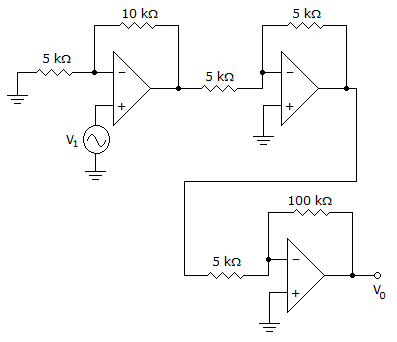–1.05 V
0.075 V
0.06 V
4.2 V
Explanation:
No answer description is available. Let's discuss.

12.
How many op-amps are required to implement this equation?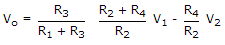2
3
4
1
Explanation:
No answer description is available. Let's discuss.

13.
Calculate the output voltage if V1 = V2 = 700 mV.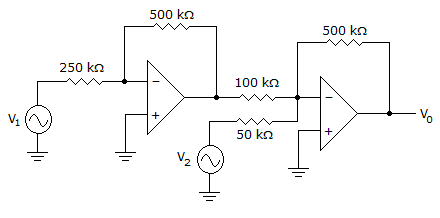0 V
–12 V
12 V
–8 V
Explanation:
No answer description is available. Let's discuss.

14.
Calculate the cutoff frequencies of a bandpass filter with R1 = R2 = 5 k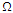and C1 = C2 = 0.1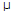F.
fOL = 318.3 Hz, fOH = 318.3 Hz
fOL = 636.6 Hz, fOH = 636.6 Hz
fOL = 318.3 Hz, fOH = 636.6 Hz
fOL = 636.6 Hz, fOH = 318.3 Hz
Explanation:
No answer description is available. Let's discuss.

15.
Calculate the output voltage if V1 = 300 mV and V2 = 700 mV.0 V
–12 V
12 V
–4 V